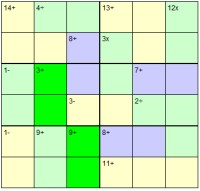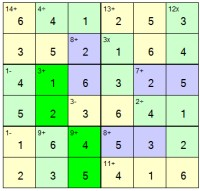Sat, 28 May 2022

# Calcudoku

Calcudoku Instructions:
Here's how to play Calcudoku:
Place the numbers from 1 - n once each in each row and column. Additionally bold-lined regions contain a variety of mathematical sums, and the values of the digits in those bold-lined regions must match the value given in each box given the mathematical operation listed. Numbers MAY repeat within these bold-lined regions.

The operations allowed are: addition, subtraction, multiplication and division.

Most confusion for players arises with non-commutative operations (subtraction and division). In these instances one must subtract (or divide) the smaller numbers from the largest one in a region. See below for example sums.

First there was sudoku. And then a short-while later came killer sudoku, which made use of the fact that one is placing numbers in the grid, by using their numerical values and splitting the grid into cages each containing a sum.

Calcudoku builds on this, but adds three other mathematical operations: subtraction, division and multiplication. The cages of killer sudoku are replaced with bold-lined regions.

Unlike killer-sudoku, there is no third region, such as the 3x3 box regions one usually finds in 9x9 killer sudoku. In addition - and this is the bit that many sudoku solvers either don't like or find it hard to get used to - one IS allowed to repeat a digit within a bold-lined region, just as long as you don't of course break the rule that a number cannot repeat in a row or column. Thus in a calcudoku puzzle where all the regions are straight horizontally or vertically (or of course if all regions are of size 2), then there is no difference between the exclusivity requirement of a killer sudoku and the fact this is not a rule in calcudoku.

When solving calcudoku puzzles, one proceeds in a very similar way to with killer sudoku, except of course performing various different operations. People find the addition and multiplication familiar enough, but some come unstuck with subtraction and division.

But the rule is simple: start with the biggest number in a region, and then divide or subtract all the other numbers from it.

Thus a region that contains 6, 3, 2 and is a minus sum comes to '1' so the symbol would be '1-'. It doesn't matter if the digits are distributed as 2, 3, 6 or 3, 6, 2 or any other combination: since the rule is to always start with the largest and proceed from there. If we were performing division, the answer here would also be 1, since 6 divided by 3 divided by 2 is 1 (as, of course, is 6 divided by 2 then divided by 3) - we just need to ensure we start the operation with the largest integer in the region.

Solving calcudokus requires one to remember that numbers can repeat within a region where that doesn't break the no-repetition-in-a-row-or-column rule, so for instance in a suitably shaped region of '3' cells, then a total of '5+' COULD be 2 + 1 + 2, unlike killer sudoku where no such repetition is allowed.

Here is a video that explains how to solve a calcudoku puzzle, if you'd like to attempt the puzzle before watching the video you can solve the Calcudoku Online now.

### Related puzzles

Arrow Sudoku, Circle Sudoku, Consecutive Sudoku, Futoshiki, Graeco-Latin Sudoku, HyperSudoku, Irregular Sudoku, Isosudoku, Killer Sudoku, Offset Sudoku, Outside Sudoku, Samurai Sudoku, Star Sudoku, Sudoku, Sudoku X, Toroidal Sudoku

Last updated: 16 Feb 2012

Sample puzzle:Sample solution:View a sample Calcudoku PDF

Play Calcudoku Puzzles:

To play calcudoku puzzles online, then there are 100 available under the name of sumdoku at the play calcudoku online section of the Puzzle Club.

If you prefer to play with paper and pencil, then you can download and then print a magazine collection of 100 calcudoku puzzles by purchasing calcudoku magazine.

Publish Calcudoku Puzzles: